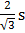# An iron ball weighing 10 kg on the earth will weigh in space (a) less than 10 kg (b) more than 10 kg (c) exactly 10 kg (d) Zero

## Question ID - 50385 :- An iron ball weighing 10 kg on the earth will weigh in space (a) less than 10 kg (b) more than 10 kg (c) exactly 10 kg (d) Zero

3537

Zero

Next Question :

The mass and the diameter of a planet are three times the respective values for the Earth. The period of oscillation of a simple pendulum on the Earth is 2s. The period of oscillation of the same pendulum on the planet would be :

 a.b. 2s c.s d.s